## Section30.3Electric Field for Spherical Symmetry

### Subsection30.3.1Spherical Symmetry of Charge Distribution

A charge distribution has a spherical symmetry if density of charge $\rho$ depends only on the distance from a center and not on the direction in space. Note that its not the shape of container of charges that determines spherical symmetry but rather the how charges are distributed as illustrated in Figure 30.3.1.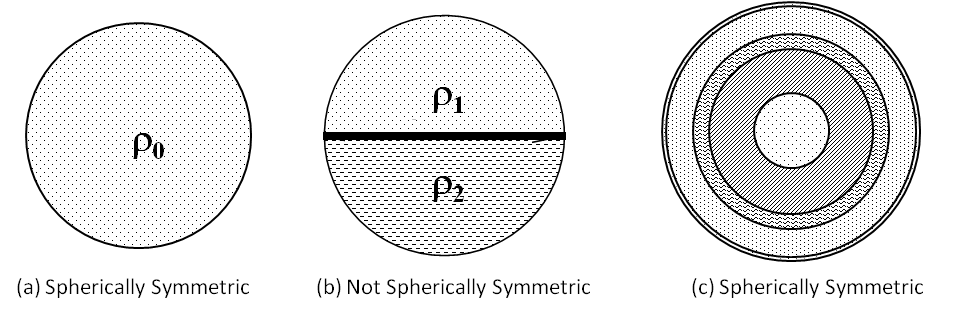Figure 30.3.1. Illustrations of spherically symmetric and non-symmetric situations. Spherical symmetry occurs only when charge density does not depend on the direction. (a) and (c) have spherical symetry, but (b) does not have spherical symmetry.

In Figure 30.3.1(a), we have a sphere of radius $R$ that is uniformly charged with constant value of $\rho_0$ everywhere. Therefore, its charge density can be written as a piecewise function of $r$ only, being same in all directions.

\begin{equation*} \rho(r,\theta, \phi) = \begin{cases} \rho_0 \amp 0\le r \le R\\ 0 \amp r \gt R. \end{cases} \end{equation*}

On the other hand, if a sphere of radius $R$ is charged so that top half of the sphere has uniform charge density $\rho_1$ and the bottom half a uniform charge density $\rho_2\ne\rho_1$ , as in Figure 30.3.1(b). Clearly, this charge density depends on the direction, and hence does not have spherical symmetry. Therefore, it is not the shape of an object but rather the shape of the charge distribution that determines whether or not a system has a spherical symmetry!

In Figure 30.3.1(c), a sphere with four different shells, each with its own uniform charge density is shown. Although this is a situation where charge density in the full sphere is not uniform, but since charge density function depends only on $r$ and not on the direction, this charge distribution does have a spherical symmetry.

### Subsection30.3.2Consequences of Symmetry

In all spherically symmetric cases, the electric field must be radially directed, either towards the center or away from the center, because there are no preferred directions in the charge distribution. Why will that be the case? Simple, for any charge that has a non-radial component, there is another charge that will have non-radial component that will cancel the non-radial component of the previous charge.

Therefore, using spherical coordinates with origin at the center of a spherical charge distribution, we can claim that electric field at a space point P located at a distance $r$ from the center can only depend on $r$ and radial unit vector $\hat u_r\text{.}$

\begin{equation} \text{Spherical symmetry:}\ \ \vec E_P = E_P(r)\hat u_r, \label{eq-spherical-sym-form-1}\tag{30.3.1} \end{equation}

Note that at this stage we do not have a formula for the electric field, we just have its direction and functional form. We will use Gauss's law to find the formula for $E_P(r)\text{.}$

Sometimes, we write the radial component $E_P(r)$ as $E_r(P)\text{,}$ or $E_r\text{,}$ or just $E\text{.}$ When $E_P \gt 0\text{,}$ the electric field at P would be pointed away from the origin, i.e., in the direction of $\hat u_r \text{,}$ and when $E_P \lt 0\text{,}$ the the electric field at P would be pointed towards the origin, i.e., in the direction of $-\hat u_r \text{.}$

### Subsection30.3.3Electric Field of a Uniformly Charged Sphere

Consider a uniformly charged sphere with charge density

\begin{equation*} \rho = \begin{cases} \rho_0 \amp 0\le r \le R\\ 0 \amp r \gt R. \end{cases} \end{equation*}

There are two types of points in this space, where we will find electric field.

1. a point $P_\text{out}$ outside the sphere, $r \gt R\text{,}$ and
2. a point $P_\text{in}$ inside the sphere, $r \le R\text{.}$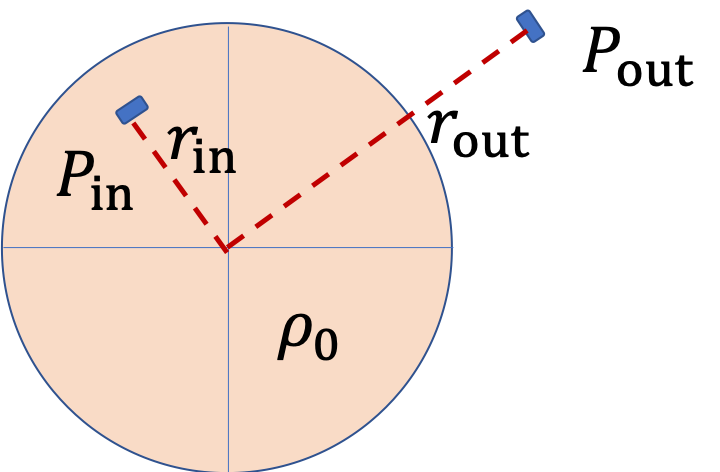We will show below that the magnitude of electric field varies with distance by two different rules, one for points inside the sphere and another for point outside the sphere.

\begin{equation*} E = \begin{cases} \dfrac{\rho_0}{3\epsilon_0}\, r \amp 0\le r \le R,\\ \dfrac{1}{4\pi\epsilon_0}\, \dfrac{q_\text{tot}}{r^2} \amp r \gt R, \end{cases} \end{equation*}

where $q_\text{tot}$ is the total charge on the sphere. Figure 30.3.2 shows a drawing of this function.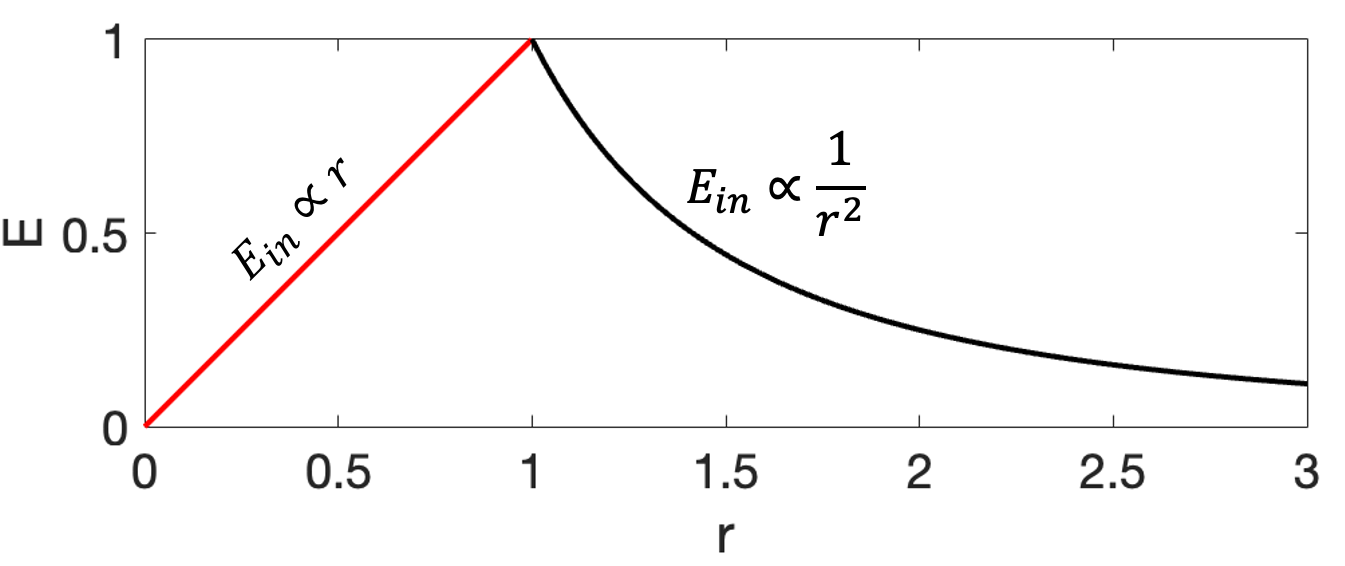Figure 30.3.2. Electric field of a uniformly charged non-conducting sphere increases inside the sphere to a maximum at the surface, and then decreases as $1/r^2\text{.}$ Here, I have scaled all constants so that $E(r=1)=1\text{.}$

#### Subsubsection30.3.3.1Electric Field at an Outside Point by Gauss's Law

Gaussian Surface and Flux

Let's call electric field at an outside point as $E_\text{out}\text{.}$ From spherical symmetry, we know that electric field at this point is radial in direction and its magnitude dependends only on the radial distance $r$ from the origin, independent of direction. To indicate this fact, we write the magnitude as a function of $r\text{.}$

\begin{equation*} E_\text{out} = E_\text{out}(r), \end{equation*}

To find electric field in the present context means we need to find the formula for this function.

In Gauss's law, electric field is inside an integral over a closed surface. So, if we want electric field at point P, we need to introduce appropriate surface that contains point P. Since electric field has same value at all points same distance as P from origin, the surface we seek is a spherical surface with center at origin. For area vectors on patches of this surface, we take normal that points from inside to outside.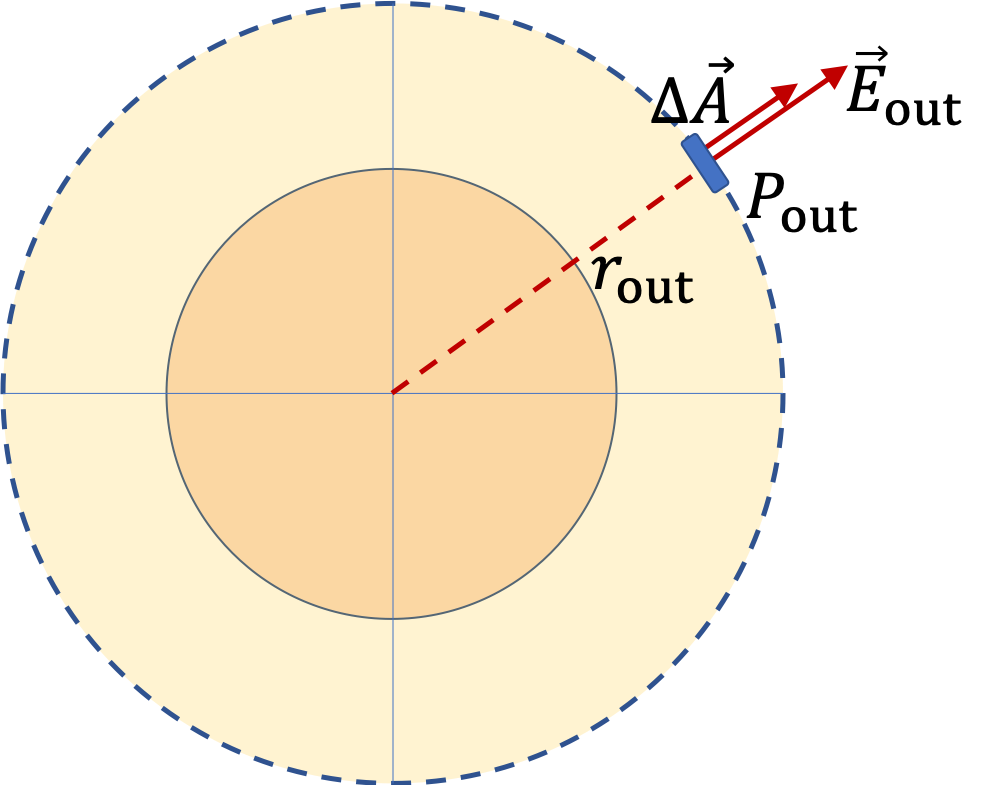Therefore, electic flux through the spherical surface will be

\begin{equation} \Phi_\text{out} = \oint_{S}\vec E\cdot d\vec A = E _\text{out}\times 4\pi r_\text{out}^2.\label{eq-gaussian-spherical-outside-1}\tag{30.3.2} \end{equation}

Enclosed Charge:

Gauss's law says that this will equal $q_\text{enc}/\epsilon_0\text{.}$ Since point $P_\text{out}$ is outside, the Gaussian surface encloses all charges in the spherical charge distribution.

\begin{equation*} q_\text{enc} = \dfrac{4}{3} \pi R^3\rho_0. \end{equation*}

Let's denote this by $q_\text{tot}\text{.}$

\begin{equation} q_\text{enc} = \dfrac{4}{3} \pi R^3\rho_0 \equiv q_\text{tot}.\label{eq-gaussian-spherical-outside-2}\tag{30.3.3} \end{equation}

Note that the volume is not the volume inside the Gaussian surface but the volume occupied by the charges.

Use Gauss's Law:

By Gauss's law we equate $\Phi_E$ from Eq. (30.3.2) to $q_\text{enc}/\epsilon_0\text{.}$

\begin{equation*} E_\text{out} \times 4\pi r_\text{out}^2 = q_\text{tot}/\epsilon_0. \end{equation*}

Solving

\begin{equation} E_\text{out} = \dfrac{1}{4\pi \epsilon_0}\, \dfrac{q_\text{tot}}{ r_\text{out}^2 }.\label{eq-gaussian-spherical-outside-3}\tag{30.3.4} \end{equation}

This is the same formula you will get if you replace spherical charge distribution by a point charge $q_\text{tot}$ at the center. That is, a spherical charge distribution produces electric field at an outside point as if it was a point charge. This is not the case at a point inside the sphere.

#### Subsubsection30.3.3.2Electric Field at an Inside Point by Gauss's Law

Let's call electric field at an inside point as $E_\text{in}\text{.}$ From spherical symmetry, we know that electric field at this point is radial in direction and magnitude just dependent on the radial distance $r$ from the origin indepdent of direction.

\begin{equation*} E_\text{in} = E_\text{in}(r), \end{equation*}

The arguments for finding this function goes similar to the way we found $E_\text{out}\text{.}$ We choose a spherical Gaussian surface that has point $P_\text{in}$ on it and has center at the origin. Now, the electric flux through this Gaussian surface will be

\begin{equation*} \Phi_\text{in} = E _\text{in}\times 4\pi r_\text{in}^2. \end{equation*}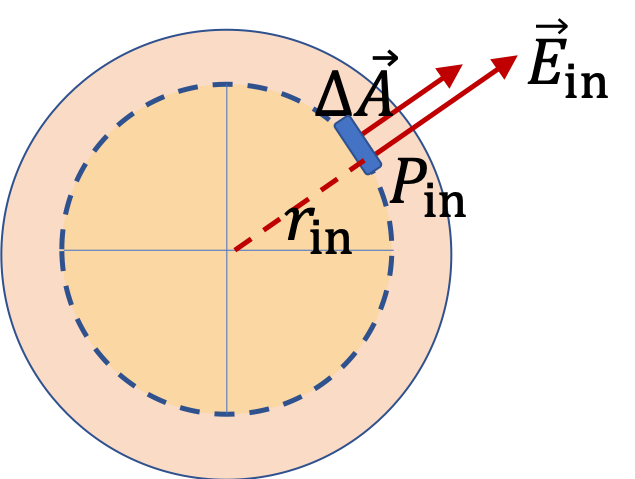But unlike the $P_\text{out}$ case, the Gaussian surface here does not include all the charges in the sphere, but only charges upto radius $r_\text{in}\text{.}$ Therefore,

\begin{equation*} q_\text{enc} = \dfrac{4}{3}\pi r_\text{in}^3\,\rho_0. \end{equation*}

Now using Gauss's law we get

\begin{equation*} E _\text{in}\times 4\pi r_\text{in}^2 = \dfrac{\dfrac{4}{3}\pi r_\text{in}^3\,\rho_0}{\epsilon_0} \end{equation*}

Therefore,

\begin{equation} E _\text{in} = \dfrac{\rho_0}{3\epsilon_0}\, r_\text{in}.\label{eq-gaussian-spherical-inside}\tag{30.3.5} \end{equation}

Notice that this says that as you move out from the center, the electric field magnitude increases as long as we are inside the sphere. Once you go outside the sphere, you will be using Eq. (30.3.4), which drops as inverse of square od the distance from the center of the sphere. Why does the electric field inside increase with distance? Clearly, this happens because you are including more and more charges within the Gaussian sphere for increasing radius points.

It is worth noting that same thing happens to gravity as well. If you move around inside Earth, force on you increases linearly from the center of Earth, but when you are outside, force decreases as inverse square of distance from the center.

Figure shows two charged concentric spherical shells. There are no charges in the space at the core, i.e., charge density, $\rho = 0,\ r\lt R_1\text{.}$ There are four distinct field points, labeled, $P_1\text{,}$ $P_2\text{,}$ $P_3\text{,}$ and $P_4\text{.}$ Find electric fields at these points.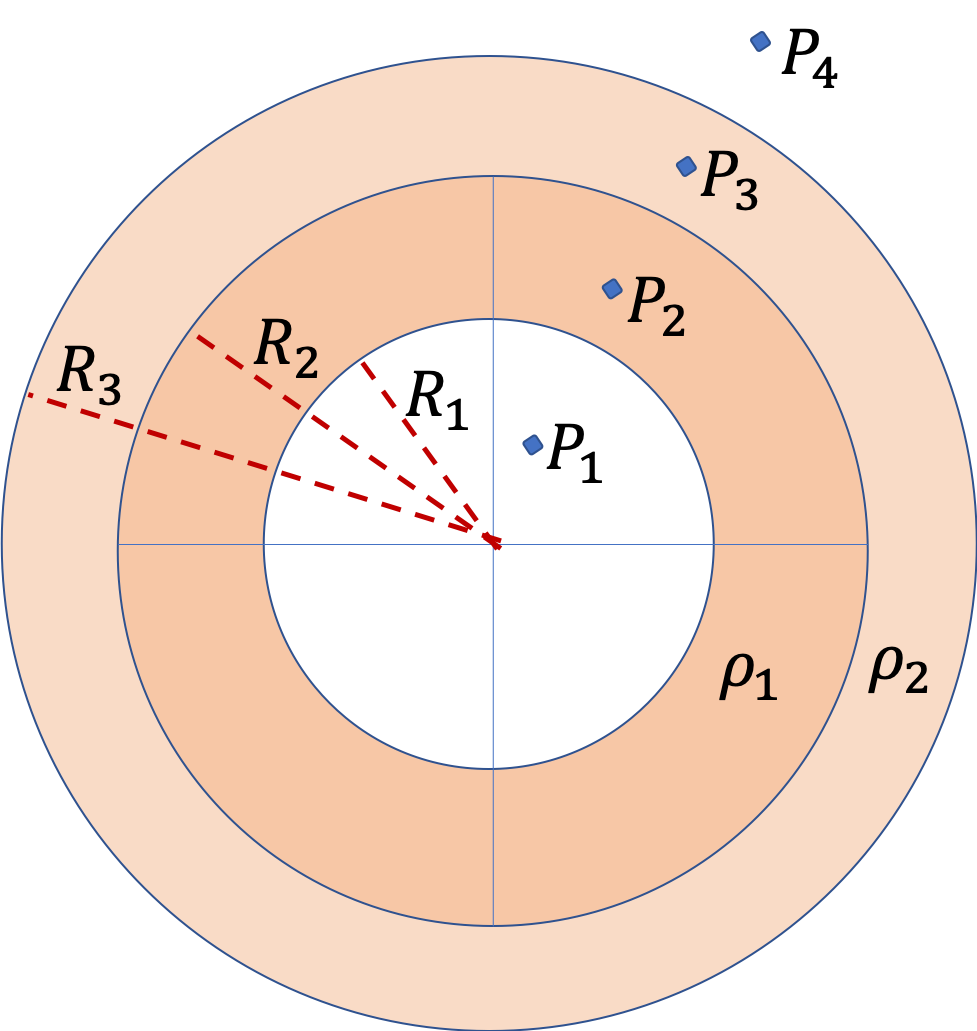Hint

Set up Gauss's law for each point.

See solution.

Solution

We first note that the charge distribution has a spherical symmetry since charge density is a function only of $r\text{,}$ the distance from the common center, and not on the direction. That means, we can use Gauss's law to find electric field rather easily.

So, if we want field at one of these points, say $P_3\text{,}$ we will imagine a spherical Gaussian surface $S_3$ that contains point $P_3\text{.}$ By spherical symmetry we already know the direction of $\vec E_3$ and the magnitude will depend on charges inside the Gaussian closing surface, which we denote by $q_\text{enc,3}\text{.}$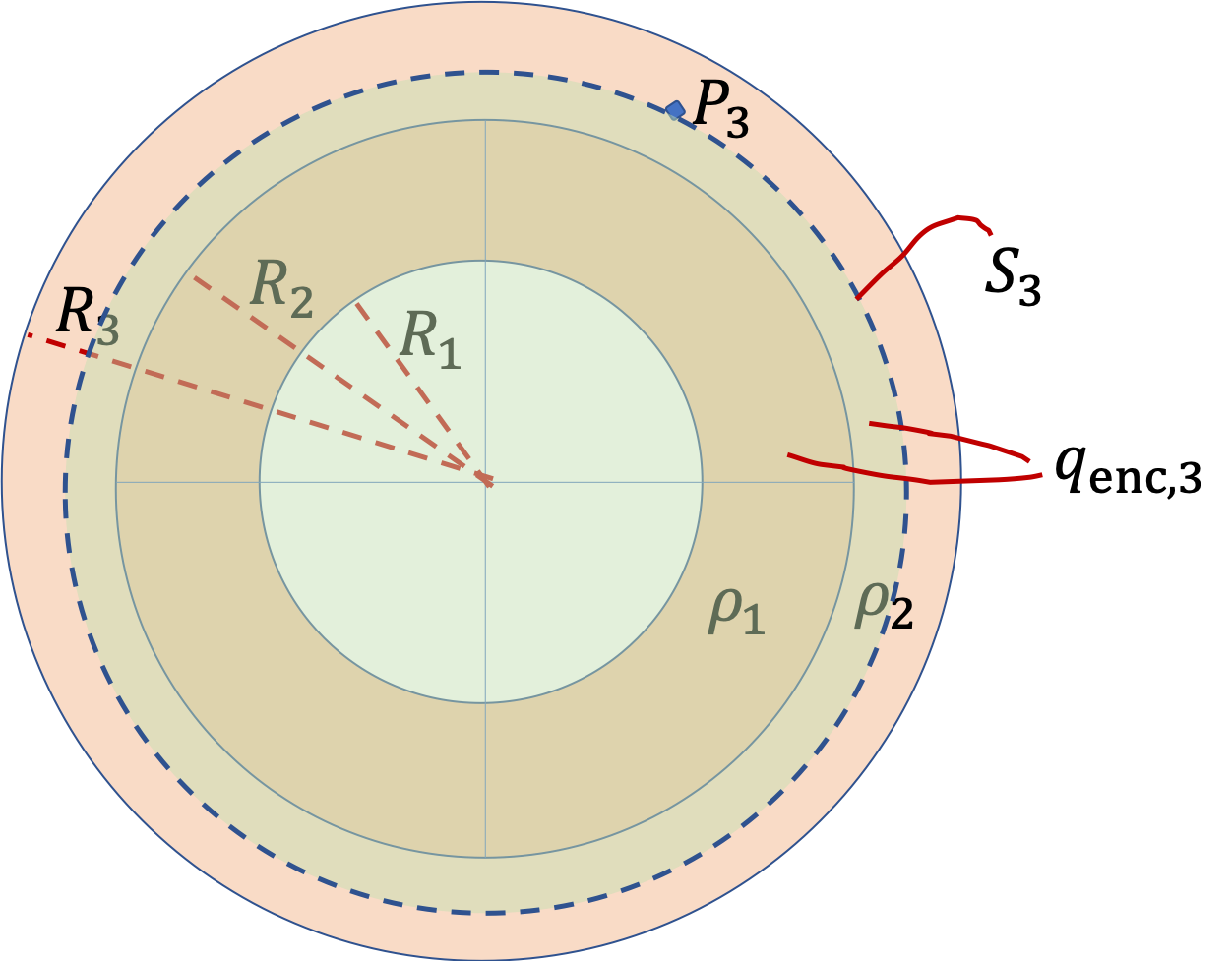\begin{equation*} E_3 = \dfrac{1}{4\pi\epsilon_0}\, \dfrac{q_\text{enc,3}}{r_3^2}. \end{equation*}

Same arguments can be applied at all four points. Let us denote the distances to the field points from the common center be $r_1\text{,}$ $r_2\text{,}$ $r_3\text{,}$ and $r_4\text{.}$ So, the only thing we need to work out the enclosed charges in each case.

\begin{align*} q_\text{enc,1} \amp = 0, \\ q_\text{enc,2} \amp = \rho_1\, \dfrac{4}{3}\pi\left(r_2^3 - R_1^3 \right), \\ q_\text{enc,3} \amp = \rho_1 \, \dfrac{4}{3}\pi\left(R_2^3 - R_1^3 \right) + \rho_2\, \dfrac{4}{3}\pi\left(r_3^3 - R_2^3 \right), \\ q_\text{enc,4} \amp = \rho_1 \, \dfrac{4}{3}\pi\left(R_2^3 - R_1^3 \right) + \rho_2\, \dfrac{4}{3}\pi\left(R_3^3 - R_2^3 \right), \end{align*}

Therefore, electric fields are radial outward if $q_\text{enc,i}$ is positive and radially inward if $q_\text{enc,i}$ is negative, and the magnitude will be

\begin{equation*} E_i = \dfrac{1}{4\pi\epsilon_0}\, \dfrac{q_\text{enc,i}}{r_i^2}. \end{equation*}

for $i=1,\ 2,\ 3, 4\text{.}$

Figure shows two charged concentric thin spherical shells. There are no charges in the space at the core, i.e., charge density, $\rho = 0,\ r\lt R_1$ or between the shells $\rho = 0,\ R_1\lt r \lt R_2\text{.}$ The two shells are uniformly charged with charge densities such that the net charge on the two shells are equal in magnitude but opposite in sign.

There are three distinct field points, labeled, $P_1\text{,}$ $P_2\text{,}$ and $P_3\text{.}$ (a) Find electric fields at these points. (b) Draw representative electric field lines for this system of charges.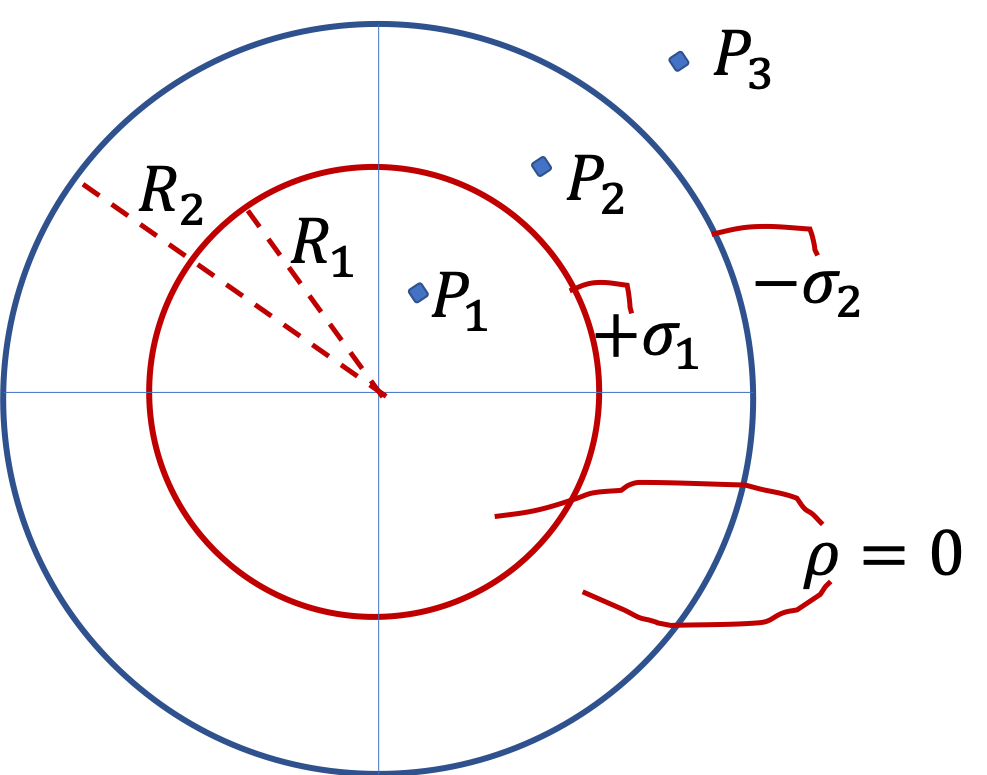How can you create this type of situation? You can start with two concentric metal shells. Place some positive charge on inner shell and same amount on the outer shell by connecting a positive terminal of a DC battery to the inner shell and the negative of the battery to the outer shell. This arrangement of metal shells is called a spherical capacitor. We will study capacitors in a future chapter.

Hint

Apply spherical Gaussian symmetry.

(a) $E_1 = 0,$ $E_2 = \dfrac{1}{4\pi\epsilon_0}\, \dfrac{4\pi R_1^2\sigma_1}{r_2^2},$ $E_3 = 0.$ (b) see the solution.

Solution 1 (a)

We first note that the charge distribution has a spherical symmetry since charge density is a function only of $r\text{,}$ the distance from the common center, and not on the direction. That means, we can use Gauss's law to find electric field rather easily.

So, if we want field at one of these points, say $P_2\text{,}$ we will imagine a spherical Gaussian surface $S_2$ that contains point $P_2\text{.}$ By spherical symmetry we already know the direction of $\vec E_2$ and the magnitude will depend on charges inside the Gaussian closing surface, which we denote by $q_\text{enc,2}\text{.}$ This surface encloses only the charge on the inner shell.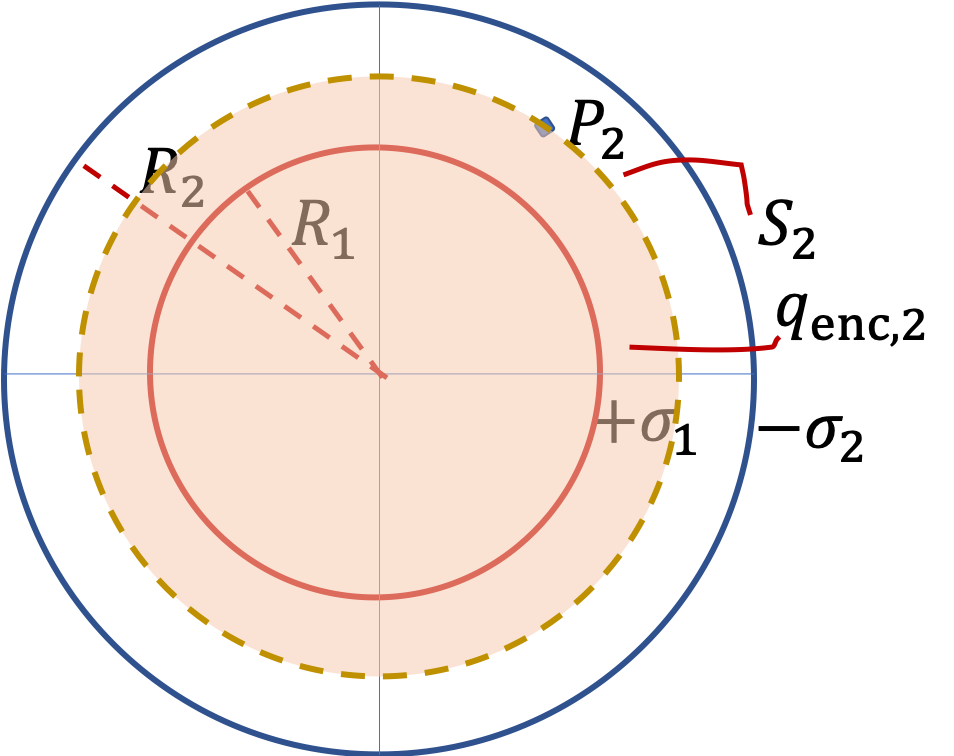\begin{align*} E_2 \amp = \dfrac{1}{4\pi\epsilon_0}\, \dfrac{q_\text{enc,2}}{r_2^2}.\\ \amp = \dfrac{1}{4\pi\epsilon_0}\, \dfrac{4\pi R_1^2\sigma_1}{r_2^2}. \end{align*}

Same arguments can be applied at all three points. Let us denote the distances to the field points from the common center be $r_1\text{,}$ $r_2\text{,}$ and $r_3\text{.}$ So, the only thing we need to work out the enclesed charges in each case.

\begin{align*} q_\text{enc,1} \amp = 0, \\ q_\text{enc,2} \amp = 4\pi R_1^2\sigma_1, \\ q_\text{enc,3} \amp = 4\pi R_1^2\sigma_1 + 4\pi R_2^2(-\sigma_2) = 0. \end{align*}

Therefore, electric fields are the stated points are

\begin{align*} E_1 \amp = 0, \\ E_2 \amp = \dfrac{1}{4\pi\epsilon_0}\, \dfrac{4\pi R_1^2\sigma_1}{r_2^2}, \\ E_3 \amp = 0. \end{align*}

That is, the only place we have non-zero electric field is in the space between the two shells. Since the inner shell is positive and outer shell negative, the electric field is radially directed from each inner shell point to a corresponding point on the outer shell.

Solution 2 (b)

The following figure shows elelectric field lines for this system.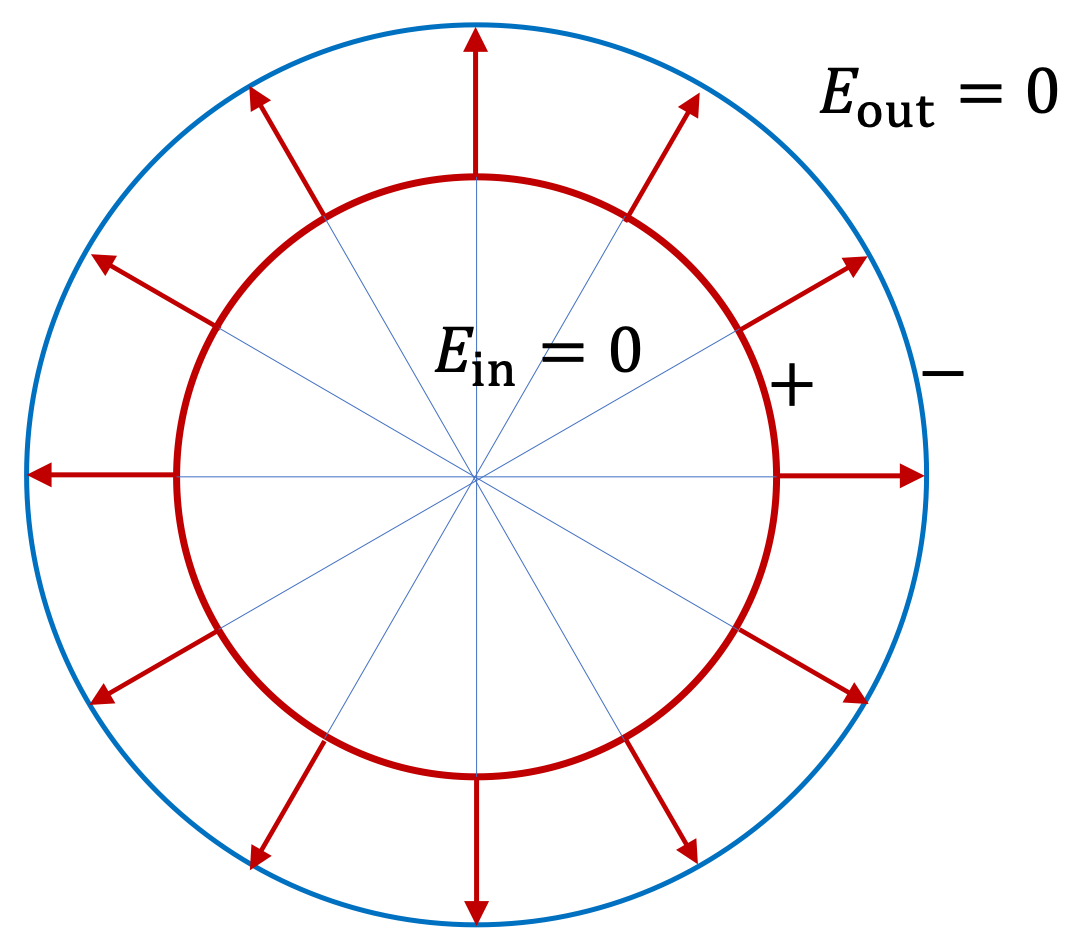A non-conducting sphere of radius $3\text{ cm}$ has a uniform charge density of $2\text{ nC/m}^3\text{.}$

1. Find the total charge contained in the sphere.
2. Find charge contained within $2\text{ cm}$ of its center.
3. Find electric flux through a spherical surface of radius $4\text{ cm}$ centered at the center of the charged sphere.
4. Find electric flux through a spherical surface of radius $2\text{ cm}$ centered at the center of the charged sphere.
5. Find electric flux through a spherical surface of radius $2\text{ cm}$ centered about a point $6\text{ cm}$ from the center of the charged sphere.
6. In the last part, you should get an answer 0. Does this mean that thre are no electric field at the location of the sphere centered about $6\text{ cm}\text{?}$ Explain.
Hint

Draw figures to guid your calculations. For electric flux, you do not need to know electric field; there is another way through Gauss's law.

(a) $2.26\times 10^{-13}\ \text{C}\text{,}$ (b) $6.70\times 10^{-14}\ \text{C}\text{,}$ (c) $2.56\times 10^{-2}\ \text{N.m}^2/\text{C}\text{,}$ (d) $7.57\times 10^{-3}\ \text{N.m}^2/\text{C}\text{,}$ (e) $0\text{,}$ (f) No, flux zero does not mean zero electric field.

Solution 1 (a)

(a) Since uniformly charged, the density of charges $\rho$ is constant. Therefore, $q_{\text{tot}} = \rho\: V\text{,}$ where $V$ is the volume of the sphere containing charges.

\begin{align*} q_{\text{tot}} \amp = \rho\: V\\ \amp = \left( 2\times 10^{-9}\right) \times \left( \frac{4}{3}\pi\ 0.03^3 \right) = 2.26\times 10^{-13}\: \text{C}. \end{align*}
Solution 2 (b)

(b) The charge contained will be in the sphere with radius 2 cm. Hence, we just scale the results of (a).

\begin{align*} \amp = q_{\text{tot}}\times \left(\frac{2\:\text{cm}}{3\:\text{cm}} \right)^3\\ \amp = 6.70\times 10^{-14}\: \text{C}. \end{align*}
Solution 3 (c)

(c) The flux will be given by Gauss's law. All charges in the sphere are enclosed by the surface at 4 cm radius.

\begin{align*} \Phi \amp = \frac{q_{\text{enc}}}{\epsilon_0} \\ \amp = \frac{2.26\times 10^{-13}\:\text{C}}{8.85\times10^{-12}\:\text{C}^2/\text{N.m}^2}\\ \amp = 2.56\times 10^{-2}\: \text{N.m}^2/\text{C}. \end{align*}
Solution 4 (d)

(d) Here only the charges inside the 2-cm radius from the center of the sphere matters. Hence,

\begin{align*} \Phi \amp = \frac{q_{\text{enc}}}{\epsilon_0}\\ \amp = \frac{6.70\times 10^{-14}\:\text{C}}{8.85\times10^{-12}\:\text{C}^2/\text{N.m}^2} \\ \amp = 7.57\times 10^{-3}\: \text{N.m}^2/\text{C} \end{align*}
Solution 5 (e)

(e) The closed surface through which flux is being calculated does not enclose any charges. Hence, $\Phi = 0\text{.}$

Solution 6 (f)

(f) No, zero electric flux does not mean zero electric field; all it means that the number of electric field lines that cross the surface in one direction are exactly equal to the number of lines cross the surface in the opposite direction. Here, since the surface is closed and is outside of any charges, every electric field line that enters in the region bounded by the surface, must come out at some point, since the lines must continue till they land on some other charge, which are all outside.

Some charge are places on a copper spherical ball of radius $2\text{ cm}$ where excess charges settle on the surface of the ball and distribute uniformly. Suppose we know that electric flux through a spherical surface of radius $30\text{ cm}$ concentric with the spherical ball is $-3\times 10^4 \text{ N.m}^2/\text{C}\text{.}$

1. What is the electric flux through a $5\text{-cm}$ radius spherical surface concentric with the copper ball?
2. What is the electric flux through a spherical surface of radius $1$ cm concentric with the copper ball?
3. What is the electric flux through a cube of side $1\text{ cm}$ side which has the center as the center of the ball?
4. What is the electric flux through a cube of side $4\text{ cm}$ that has the same center as the center of the ball.
Hint

Use Gauss's law. Make sure you understand, whether charges are enclosed within the Gaussian surface or not.

(a) $-3.0\times 10^4\ \text{N.m}^2/\text{C}\text{,}$ (b) $0\text{,}$ (c) $0\text{,}$ (d) $-3.0\times 10^4\ \text{N.m}^2/\text{C}\text{.}$

Solution 1 (a)

(a) The 5-cm spherical surface about the 2-cm spherical ball encloses same amount of charge as the 30-cm spherical surface about the same ball. Hence, $\Phi = - 3\times 10^{4}\text{ N.m}^2/\text{C}\text{.}$

Solution 2 (b)

(b) $\Phi = 0$ since no charge is enclosed within the spherical surface with radius $1\text{ cm}\text{.}$

Solution 3 (c)

(c) $\Phi = 0$ since no charge is enclosed within the cubic surface with side $1\text{ cm}\text{.}$

Solution 4 (d)

(d) The cube of side 4 cm will enclose the same amount of charge as the 30-cm spherical surface about the same ball. Hence, $\Phi = - 3\times 10^{4}\text{ N.m}^2/\text{C}\text{.}$

A small copper ball of radius $1.0\text{ cm}$ with $+1.5\text{ nC}$ on its surface is surrounded by an uncharged gold spherical shell with inner radius $2.0\text{ cm}$ and outer radius $3.0\text{ cm}\text{.}$ Due to induction, the inner surface of the gold shell develops a charge $-1.5\text{ nC}$ , uniformly spread out and the outer surface develops a charge $+1.5\text{ nC}\text{,}$ also uniformly spread over the surface.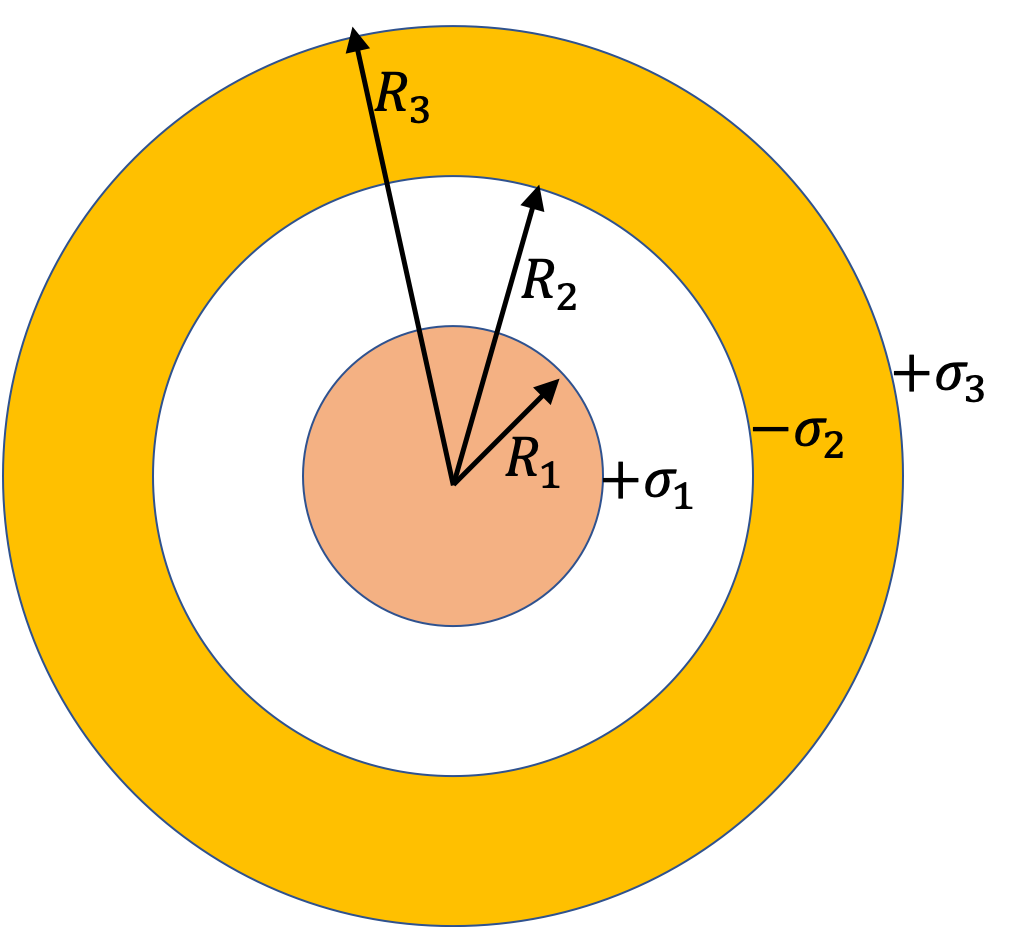1. Determine the electric flux through the spherical surfaces of radii: (i) $0.5\text{ cm}\text{,}$ (ii) $1.5\text{ cm}\text{,}$ (iii) $2.5\text{ cm}\text{,}$ and (iv) $4.0\text{ cm}$ concentric with the gold shell.
2. Find the magnitude of electric field at three points (i) $P_1$ at a distance $0.5\text{ cm}$ from the common center, (ii) $P_2$ at a distance $1.5\text{ cm}$ from the common center, (iii) $P_3$ at a distance $2.5\text{ cm}$ from the common center, (iv) $P_4$ at a distance $4.0\text{ cm}$ from the common center.
3. Draw electric field lines.
Hint 1 (a)

For flux through closed surface, we can use Gauss's law, and get it from information on charge.

Hint 2 (b)

Use Gauss's law.

Hint 3 (c)

Use the electric field you worked out.

(a) (i) $0\text{,}$ (ii) $170\text{ N.m}^2\text{/C}\text{,}$ (iii) $0\text{,}$ (iv) $170\text{ N.m}^2\text{/C}\text{,}$ (b) (i) $0\text{,}$ (ii) $60,125\text{ N/C}\text{,}$ (iii) $0\text{,}$ (iv) $8,455\text{ N/C}\text{.}$

Solution 1 (a)

Let $r$ denote distance of a point from the common center.

(i) No charges are inside the volume enclosed by the closed surface at $r=0.5\text{ cm}\text{.}$ Therefore, by Gauss's law, flux will be zero.

(ii) Enclosed charge is equal to all the charges on the copper ball. That means, $q_\text{enc} = +1.5\text{ nC}\text{.}$ Therefore, by Gauss's law, flux will be

\begin{equation*} \Phi = \dfrac{q_\text{enc}}{\epsilon_0} = 170\text{ N.m}^2\text{/C}. \end{equation*}

(iii) Enclosed charge is equal to sum of the charges on the copper ball and the charges on the inner surface of the gold shell. That means, $q_\text{enc} = +1.5\text{ nC} - 1.5\text{ nC} = 0\text{.}$ Therefore, by Gauss's law, flux will be zero.

(iv) Enclosed charge is equal to sum of the charges on the copper ball, the charges on the inner surface of the gold shell, and the charges on the outer surface of the gold shell. That means, $q_\text{enc} = +1.5\text{ nC} - 1.5\text{ nC} + 1.5\text{ nC} = + 1.5\text{ nC}\text{.}$ Therefore, by Gauss's law, flux will be

\begin{equation*} \Phi = 170\text{ N.m}^2\text{/C}. \end{equation*}
Solution 2 (b)

Let $r$ denote the distance from center. We note that charge distribution on the three locations maintain the spherical symmetry of the charge distribution. Therefore, all the Gaussian surfaces will be sperical with center same as the center of the charge distribution.

(i) We use a spherical Gaussian surface of radius $r_1 = 0.5\text{ cm}\text{.}$ From the spherical symmetry, Gauss's law for this surface gives

\begin{equation*} 4\pi r_1^2 E_1 = \dfrac{q_\text{end}}{\epsilon_0} = 0. \end{equation*}

Therefore,

\begin{equation*} E_1 = 0. \end{equation*}

(ii) Applying Gauss's law to a spherical Gaussian surface through the point under consideration gives

\begin{equation*} 4\pi r_2^2 E_2 = 170\text{ N.m}^2\text{/C}. \end{equation*}

With $r_2 = 1.5\text{ cm}$ we get

\begin{equation*} E_2 = 60,125\text{ N/C}. \end{equation*}

(iii) Same logic as in (b)(i) we get $E_3 = 0\text{.}$

(iv) Same logic as in (b)(ii) will lead to a simlar formula in which distance will now be $4.0\text{ cm}\text{.}$

\begin{equation*} E_4 = \dfrac{170\text{ N.m}^2\text{/C}}{ 4\pi 0.04^2} = 8455\text{ N/C}. \end{equation*}
Solution 3 (c)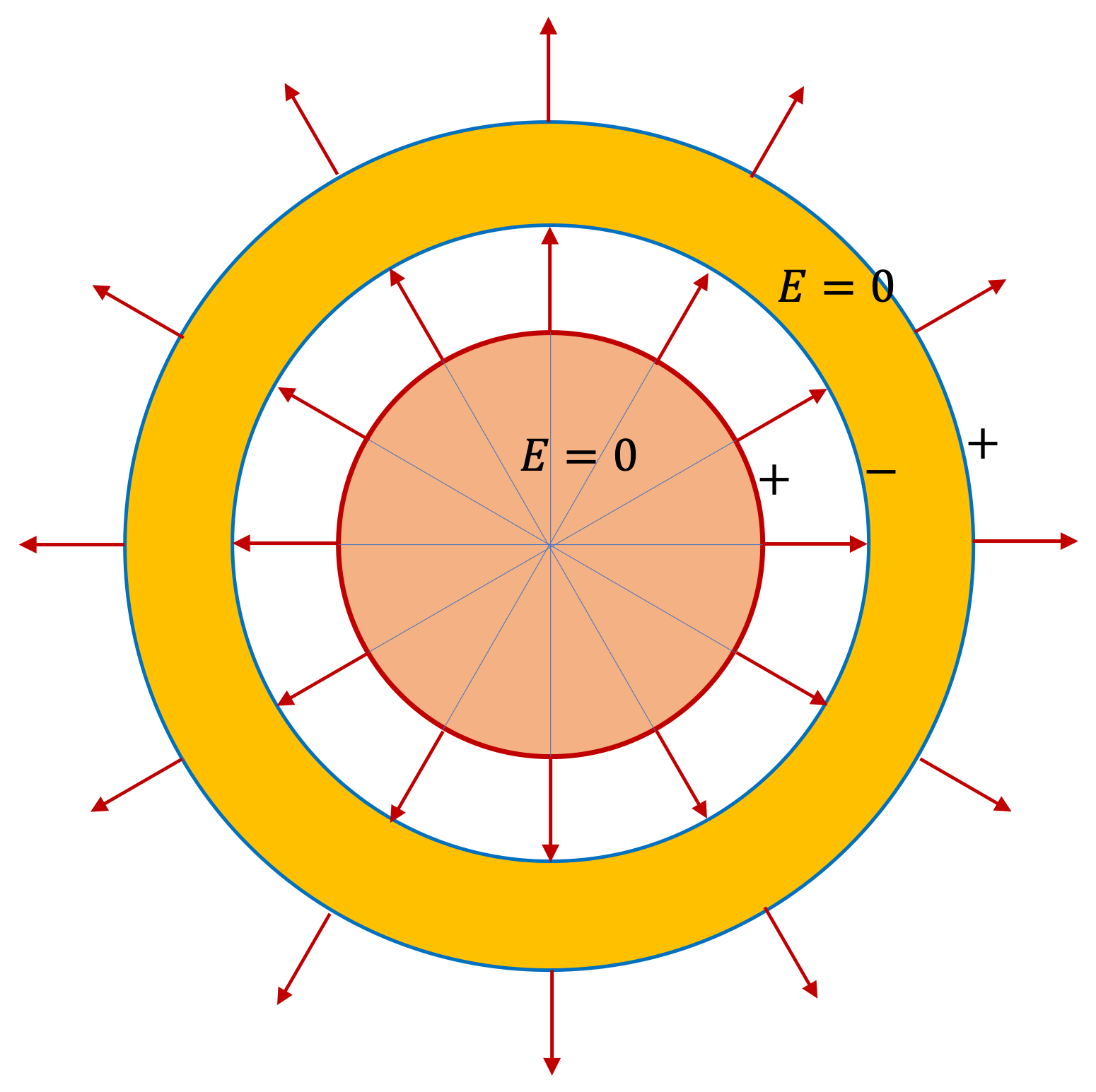A 3D printer is used to deposit charges on a nonconducting sphere. The sphere grows from a radius $R_1$ to a radius $R_2$ such that the charge density varies as

\begin{equation*} \rho = \rho_0 \frac{a}{r}, \ \ R_1\le r\le R_2, \end{equation*}

where $\rho_0$ and $a$ are some constants. Find electric field at (a) a point outside the sphere, and (b) a point inside the spherical shell grown by the printer.

Hint

(a) and (b): You will need to integrate to get enclosed charge.

(a) $\frac{\rho_0 a}{2\epsilon_0} \frac{R_2^2 - R_1^2}{r_\text{out}^2} \text{,}$ (b) $\frac{\rho_0}{2\epsilon_0}\left( 1 - \frac{R_1^2}{r_\text{in}^2} \right) \text{.}$

Solution 1 (a)

(a) Because of spherical symmetry, the direction of the field will be radial. Since field point P is outside the distribution, Gaussian surface encloses all charges and from Gauss's law we get the following for magnitude $E_\text{out}\text{.}$

\begin{equation*} E_\text{out} \times 4\pi r_\text{out}^2 = \frac{q_\text{total}}{\epsilon_0}. \end{equation*}

To obtain the total charge we just need the $r$ integral from $r=R_1$ to $r=R_2\text{,}$ the radius of the distribution.

\begin{align*} q_\text{total} \amp = \int_{R_1}^{R_2} \rho\ 4\pi r^2 dr \\ \amp = 4\pi \rho_0 a \int_{R_1}^{R_2} r dr\\ \amp = 2\pi \rho_0 a \left( R_2^2 - R_1^2\right) . \end{align*}

Therefore, electric field will be

\begin{equation*} E_\text{out} = \frac{\rho_0 a}{2\epsilon_0} \frac{R_2^2 - R_1^2}{r_\text{out}^2}. \end{equation*}
Solution 2 (b)

(b) For a point inside the sphere, Gaussian surface will not include all charges, just the charges from $r=R_1$ upto the Gaussian surface at $r=r_\text{in}\text{.}$ Let's denote $r=r_\text{in}$ for the radius of this surface. We get,

\begin{equation*} E_\text{in} \times 4\pi r_\text{in}^2 = \frac{q_\text{enc}}{\epsilon_0}, \end{equation*}

with $q_\text{enc}$

\begin{equation*} q_\text{enc} = \int_{R_1}^{r_\text{in}} \rho\ 4\pi r^2 dr. \end{equation*}

This gives

\begin{equation*} q_\text{enc} = 2\pi\rho_0 a \left( r_\text{in}^2 - R_1^2 \right). \end{equation*}

Therefore,

\begin{equation*} E_\text{in} = \frac{\rho_0}{2\epsilon_0}\left( 1 - \frac{R_1^2}{r_\text{in}^2} \right). \end{equation*}